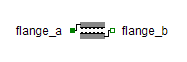Vector Norm - MapleSim Help

Vector Norm

Compute the 2-norm of a real vectorDescription The Vector Norm component computes the 2-norm of a vector.
 Equations $y=\sqrt{\sum _{j=1}^{n}{u}_{j}^{2}}$

Connections

 Name Description Modelica ID $u$ Real input vector u $y$ Real output vector y

Parameters

 Name Default Units Description Modelica ID $n$ $3$ dimension of input vector n

 Modelica Standard Library The component described in this topic is from the Modelica Standard Library. To view the original documentation, which includes author and copyright information, click here.